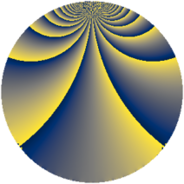# Properties

 Label 385.2.bdLevel $385$ Weight $2$ Character orbit 385.bd Rep. character $\chi_{385}(12,\cdot)$ Character field $\Q(\zeta_{12})$ Dimension $160$ Newform subspaces $2$ Sturm bound $96$ Trace bound $8$

# Related objects

## Defining parameters

 Level: $$N$$ $$=$$ $$385 = 5 \cdot 7 \cdot 11$$ Weight: $$k$$ $$=$$ $$2$$ Character orbit: $$[\chi]$$ $$=$$ 385.bd (of order $$12$$ and degree $$4$$) Character conductor: $$\operatorname{cond}(\chi)$$ $$=$$ $$35$$ Character field: $$\Q(\zeta_{12})$$ Newform subspaces: $$2$$ Sturm bound: $$96$$ Trace bound: $$8$$ Distinguishing $$T_p$$: $$2$$

## Dimensions

The following table gives the dimensions of various subspaces of $$M_{2}(385, [\chi])$$.

Total New Old
Modular forms 208 160 48
Cusp forms 176 160 16
Eisenstein series 32 0 32

## Trace form

 $$160q - 24q^{8} + O(q^{10})$$ $$160q - 24q^{8} + 24q^{15} + 72q^{16} - 36q^{17} - 16q^{18} - 56q^{21} - 8q^{23} - 76q^{28} - 16q^{30} + 24q^{31} + 64q^{32} - 12q^{35} - 208q^{36} + 12q^{38} - 48q^{40} + 88q^{42} + 48q^{43} + 36q^{45} - 24q^{46} - 72q^{47} - 8q^{50} - 108q^{52} - 24q^{53} + 24q^{56} - 120q^{57} + 24q^{58} + 48q^{60} + 24q^{61} - 104q^{63} + 48q^{65} - 8q^{67} - 144q^{68} + 132q^{70} + 16q^{71} + 52q^{72} + 96q^{73} + 48q^{75} - 72q^{78} + 108q^{80} + 48q^{81} + 72q^{82} + 48q^{85} - 40q^{86} - 36q^{87} + 24q^{88} - 112q^{91} - 40q^{92} - 20q^{93} + 8q^{95} + 168q^{96} + 48q^{98} + O(q^{100})$$

## Decomposition of $$S_{2}^{\mathrm{new}}(385, [\chi])$$ into newform subspaces

Label Dim. $$A$$ Field CM Traces $q$-expansion
$$a_2$$ $$a_3$$ $$a_5$$ $$a_7$$
385.2.bd.a $$80$$ $$3.074$$ None $$0$$ $$0$$ $$0$$ $$0$$
385.2.bd.b $$80$$ $$3.074$$ None $$0$$ $$0$$ $$0$$ $$0$$

## Decomposition of $$S_{2}^{\mathrm{old}}(385, [\chi])$$ into lower level spaces

$$S_{2}^{\mathrm{old}}(385, [\chi]) \cong$$ $$S_{2}^{\mathrm{new}}(35, [\chi])$$$$^{\oplus 2}$$